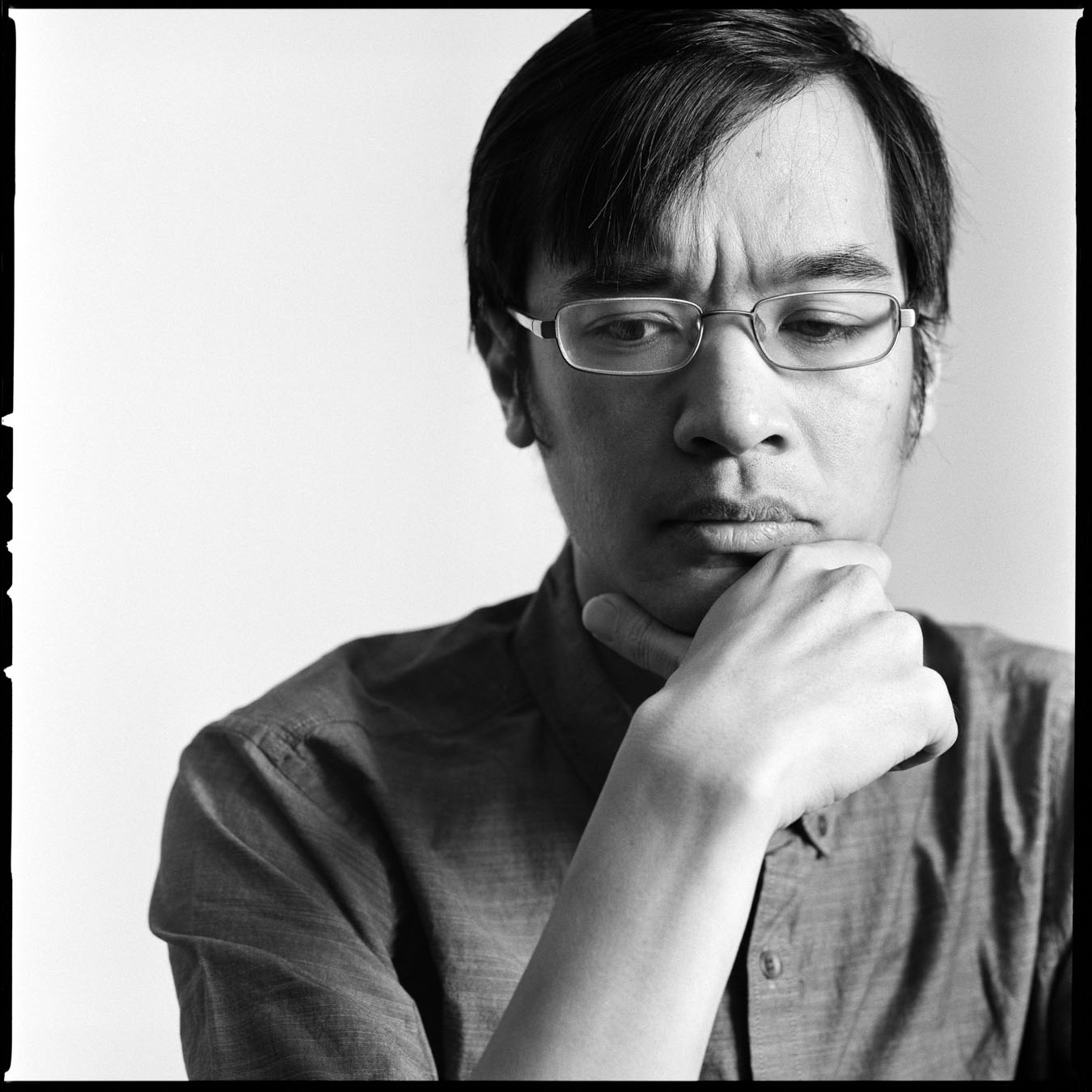# Laureates

### Terence Tao

University of California, Los Angeles

#### 2015 Breakthrough Prize in Mathematics

For numerous breakthrough contributions to harmonic analysis, combinatorics, partial differential equations, and analytic number theory.

#### The Science

Terence Tao has made important contributions to a very wide range of mathematical fields, but has perhaps made the most impact in number theory. Number theory is the study of integers, and prime numbers play a particularly central role: in the universe of numbers, primes are the atoms. Alone and with collaborators, Tao has solved several longstanding problems related to prime numbers. For example, he proved that every odd integer above 1 can be built from adding together five prime numbers or less; and that there are equally spaced progressions of primes (such as 3, 7, 11), of any desired length, to be found within the infinite series of integers.